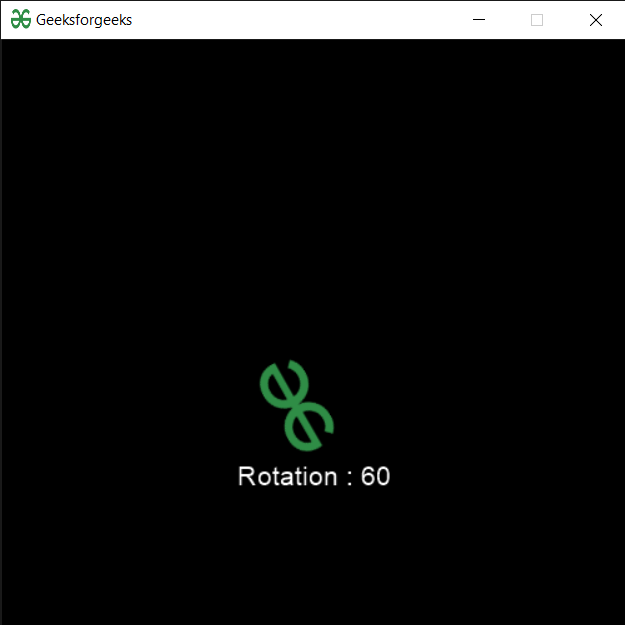Related Articles

# PYGLET – Sprite Rotation

• Last Updated : 23 Sep, 2020

In this article we will see how we can access rotation of the sprite in PYGLET module in python. Pyglet is easy to use but powerful library for developing visually rich GUI applications like games, multimedia etc. A window is a “heavyweight” object occupying operating system resources. Windows may appear as floating regions or can be set to fill an entire screen (fullscreen). A sprite is an instance of an image displayed on-screen. Multiple sprites can display the same image at different positions on the screen. Sprites can also be scaled larger or smaller, rotated at any angle and drawn at a fractional opacity. Image is loaded with the help of image module of pyglet. Sprite rotation is clockwise rotation of the sprite, in degrees. The sprite image will be rotated about its image’s (anchor_x, anchor_y) position.

We can create a window and sprite object with the help of commands given below

```# creating a window
window = pyglet.window.Window(width, height, title)

# creating a sprite object
sprite = pyglet.sprite.Sprite(img, x, y)
```

In order to create window we use `rotation` attribute with sprite object

Syntax : sprite.rotation

Argument : It takes no argument

Return : It returns float value

Below is the implementation

 `# importing pyglet module ``import` `pyglet ``import` `pyglet.window.key as key``   ` `# width of window ``width ``=` `500``   ` `# height of window ``height ``=` `500``   ` `# caption i.e title of the window ``title ``=` `"Geeksforgeeks"``   ` `# creating a window ``window ``=` `pyglet.window.Window(width, height, title) ``   ` `# text  ``text ``=` `"Welcome to GeeksforGeeks"``  ` `# creating label with following proeprties``# font = cooper``# position = 250, 150``# anchor position = center``label ``=` `pyglet.text.Label(text, ``                          ``font_name ``=``'Cooper'``, ``                          ``font_size ``=` `16``, ``                          ``x ``=` `250``,  ``                          ``y ``=` `150``, ``                          ``anchor_x ``=``'center'``,  ``                          ``anchor_y ``=``'center'``)`` ` ` ` `# creating a batch ``batch ``=` `pyglet.graphics.Batch()`` ` `# loading geeksforgeeks image``image ``=` `pyglet.image.load(``'gfg.png'``)`` ` ` ` ` ` `# creating sprite object``# it is instance of an image displayed on-screen``sprite ``=` `pyglet.sprite.Sprite(image, x ``=` `200``, y ``=` `230``)``   ` `# on draw event ``@window``.event ``def` `on_draw(): ``       ` `    ``# clear the window ``    ``window.clear() ``       ` `    ``# draw the label``    ``label.draw() ``     ` `    ``# draw the image on screen``    ``sprite.draw()``       ` `# key press event     ``@window``.event ``def` `on_key_press(symbol, modifier): ``   ` `    ``# key "C" get press ``    ``if` `symbol ``=``=` `key.C: ``         ` `        ``# printng the message``        ``print``(``"Key : C is pressed"``)``         ` `# image for icon ``img ``=` `image ``=` `pyglet.resource.image(``"gfg.png"``) `` ` `# setting image as icon ``window.set_icon(img) `` ` `# accessing rotation of the sprite``value ``=` `sprite.rotation ``=` `60`` ` `# creating text from value``text ``=` `"Rotation : "` `+` `str``(value)`` ` `# setting text to the label``label.text ``=` `text``    ` `# start running the application ``pyglet.app.run() `

Output :Attention geek! Strengthen your foundations with the Python Programming Foundation Course and learn the basics.

To begin with, your interview preparations Enhance your Data Structures concepts with the Python DS Course. And to begin with your Machine Learning Journey, join the Machine Learning – Basic Level Course

My Personal Notes arrow_drop_up